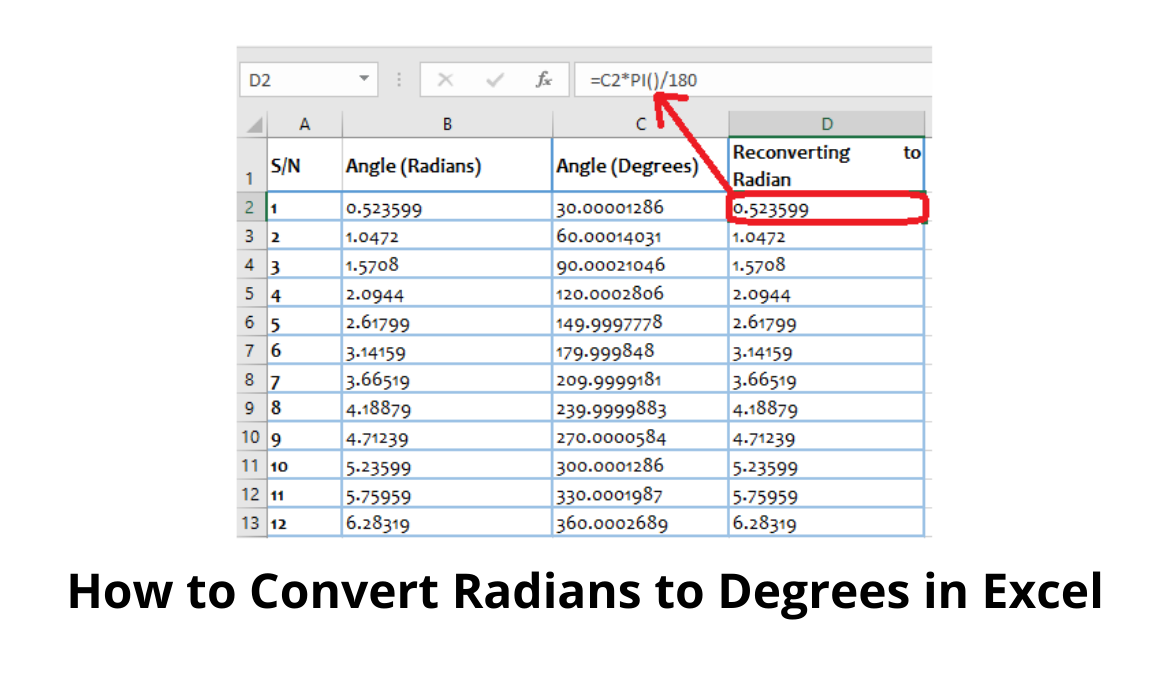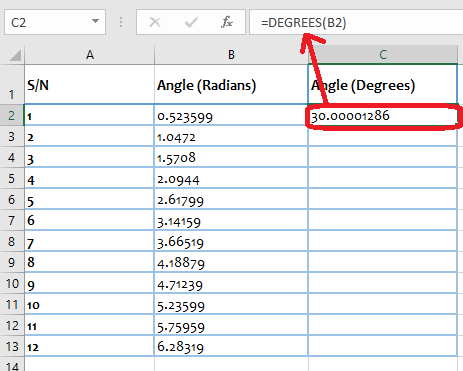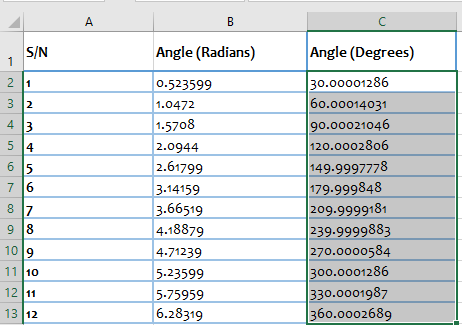# How to Convert Radians to Degrees in ExcelExcel has incorporated, some trigonometric capabilities that can help you effortlessly calculate the cosine, sine, or tangent of angles.

You would agree with me that solving trigonometric problems requires detailed attention.

More important is ensuring that the right unit of measurement is used during calculation and when displaying results.

Should the reverse be the case (having the wrong unit of measurement), it invalidates the correctness of your calculation.

Generally, there are three units of measure for angles and they are:

• Degrees
• Revolution

The default unit of measure in Excel for trigonometric problems is the Radians and it is important to be able to convert between any of the three units.

In today’s tutorial, we will learn how to convert Radians to Degrees in excel.

## Methods to Convert Radians to Degrees in Excel

We will achieve this by utilizing three methods:

• Using a function
• Using VBA
• Using Formula

To aid your understanding in this tutorial, let us quickly define some key terms

• Function: This is a pre-established formula that performs calculations by using specific values, called arguments, in a particular order E.g., PI, DEGREE, RADIANS
• VBA – This is an acronym for Visual Basic Application. It is an event-driven programming language
• Sub: This is short for Subroutine. A subroutine is primarily a piece of code that executes a specific task described in the code
• Dim: This is an acronym for Declare in Memory. It is used to declare a variable to a specific data type
• Set – This is a statement that is used to assign a value to an object
• Radius: This is a straight line from the center of a circle to any part of the circumference

The table below shows a series of angles in radian.

Our task today will be to convert this series of angles to degrees.

 S/N Angle (Radians) 1 0.523599 2 1.0472 3 1.5708 4 2.0944 5 2.61799 6 3.14159 7 3.66519 8 4.18879 9 4.71239 10 5.23599 11 5.75959 12 6.28319

## Using a function

There exist various trigonometric functions in excel that can be used to carry out various tasks.

In this case, we will be utilizing the DEGREES function.

This function was specifically built to change angles in radians to degrees.

The syntax of the DEGREES function is:

DEGREES (angle)

Here the argument ‘angle’ refers to the angle in degrees to be changed to radians.

You can specify the angle size in radians (eg. 0.4563) or a specific cell reference where the angle size resides in radians.

For example, if you wish to change the value 0.432 radians to degrees, all you need do is to simply type:

DEGREES (0.432)

If the angle size or value is stored in cell B3, you could reference the location by:

DEGREES (B3)

When you press the ENTER key in both cases the result displayed will be the same.

To convert the series of angles in our sample table to the degree equivalent, we will follow the outlined steps shown below:

Step 1: Navigate to the Cell where you wish to display your converted angle and Select it. In our example, it will be Cell C2

Step 2: Type the ‘equal to’ sign ‘=’

Step 3:  Type in the words ‘DEGREES

Step 4: Input the opening bracket symbol ‘(‘

Step 5: Select the cell which contains the angle you wish to convert. In our example, this will be cell B2.

Step 6: Close the bracket using the closing parenthesis symbol ‘)’

Step 7: Press the ENTER key on your keyboard.

Step 8: The degrees equivalent of the selected reference cell will be displayed.Step 9: Select the cell where the result was displayed (in our example, C2) You will notice a square at the bottom right of the cell. This square is called the ‘fill handle’

Step 10: Copy the formula to the rest of the cells in the column by double-clicking the fill handle. Alternatively, you can choose to drag down the fill handle to achieve the same effect.You will discover that the precise result in degree is displayed.

Sometimes the result is displayed in several decimal places.

If we wish to round up the result, we will employ the ROUND function.

However, if we are comfortable with the way the answers are displayed, you may ignore the steps below.

This function has a syntax of

ROUND(number, num_digits)

Where:

• Number – This is the number that you want to round
• num_digits – This is the number of digits to which you want to round the number argument to. For example, if you want the number argument to be rounded to zero (0) decimal places.

Thus, if we have to apply the ROUND function on the result displayed in Column ‘C’ we will simply do the following

• Navigate to the Cell where you wish to display your rounded angle and select it. In our example, it will be Cell D2
• Type the ‘equal to’ sign ‘=’
• Type in the words ‘ROUND
• Input the opening bracket symbol ‘(‘
• Select the cell which contains the angle you wish to convert. In our example, this will be cell C2.
• Type in a comma ‘,’
• Input the num_digits (that is the number of decimal places you wish to round the number argument to). In our example, the num_digits has been set to 0.
• Close the bracket using the closing parenthesis symbol ‘)’
• Press the ENTER key on your keyboard.
• The rounded number of the selected reference cell will be displayed.NOTE:

• The number is rounded to the specified number of decimal places if the num_digits is greater than zero (0)
• The number is rounded to the nearest integer if the num_digits is zero (0)
• The number is rounded to the left of the decimal point if the num_digits is less than zero (0)
• Use the ROUNDUP function to round up a number (that is to round away from zero)
• Use the ROUNDDOWN function to round down a number (that is to round toward zero)

If you desire to convert these values (in degrees)  back to the radians equivalent, you need to use the RADIANS function in excel

The syntax for the RADIANS function is:

Hence, if cell C2 contains the value in degrees that you want to convert to radians, you need to type:

And to replicate this across a column, simply hold and drag the fill handle across the desired range.

## Using VBA

VBA allows you to easily automate various activities in Excel.

This is done by writing a program from the backend.

I know this sounds like a programmer’s task, but trust me when I say it’s very easy.

Firstly, we need to be sure that the “Developer” Tab in your excel ribbon is activated.

If this isn’t the case, simply

• Go to File
• Click on Options from the excel dialog box
• Click on the Customize Ribbon in the left pane
• Check the Developers option on the right pane
• Click OK.

Now let’s get started with VBA!

Here I will show you have to write a program to convert a range of cells from Radians to Degrees and Vice versa.

Step 1: Click on the Developer tab

Step 2: Select the Visual Basic Icon on the LeftThe VBA Window opens.

Step 3: Click Insert and Select ModuleA blank Module window is created.

Step 4: Copy and paste the code below

```Sub Convert_From_Radians_to_Degrees()
Dim myrng As Range
Dim cell As Range
Set myrng = Application.Selection
For Each cell In myrng
cell.Offset(0, 1).Value = Application.WorksheetFunction.Degrees(cell.Value)
Next
End Sub```Let me quickly explain what we are trying to achieve with this code

This is a For Loop program.

During the code execution, an analysis of all cells within the range selection is carried out.

For every cell analyzed, it converts the value to a degree equivalent and displays the result in the next column.

Step 5:   Close your VBA window

Step 6: Navigate to the worksheet and select the range of cells containing the text you wish to convert (In our example, this is B2:B13)

Note: Ensure that the next column after the selection is blank.

Step 7: Click on the Developer tab

Step 8: Select the Macros Icon on the Left

Step 9: A Micros dialog box pops out

Step 10: In the “Macros name” field, Select the Subroutine name you just created on your VBA (In our example, the subroutine is ‘Convert_From_Radians_to_Degrees’)

Step 11: Click on the Run button.

You will notice that the converted values occupy the next column.

If you desire to convert these values (in degrees)  back to the radians equivalent, you copy the code below and run.

```Sub Convert_From_Radians_to_Degrees()
Dim myrng As Range
Dim cell As Range
Set myrng = Application.Selection
For Each cell In myrng
Next
End Sub```

This code will convert the selected range and converts its values to its radian equivalent.

## Using a Formula

This formula uses the Pi (π) function.

Let’s refresh our basic mathematic memory!

Imagine that you have a circle.

If we stretch two radii from the middle of the circle to the circumference so that a sector is formed (As shown below).By definition, the angle between the two radii ‘l’ (that is the angle in the small sector) is equal to a radian.

Let us call the other angle (that is the angle in the bigger sector Θ radians)

Θ = ‘2 x π x r’  (units)

Cross multiplying:

Θ x r =  2 x π x r x 1 radian

Θ  = ( 2 x π x r x 1 radian ) / r

If the r from the denominator cancels r from the numerator we will be left with

Recall that

Θ = 360 degrees

Hence

π radian = 180 degrees —– This is our base

Therefore, if the angle is in degrees, multiply it by π /180° to convert it to radians.

And if the angle is in radian multiply it by 180° / π to convert it to degrees

In Excel, π is denoted with Pi.

This is a function in excel and it returns a constant accurate to 15 digits.

With Excel, the above formula can be rewritten as:

(number) * Pi()/180 —– to convert from degrees to radians

and

(number) * 180/Pi() —– to convert from radians to degrees

Now let us see how to apply this formula in excel.

From our sample table, if we have to populate Column C with the degree equivalent of the series of angles recorded in Column C, we will follow the steps captured below:

Step 1: Navigate to the Cell where you wish to display your converted angle and Select it. In our example, it will be Cell C2

Step 2: Type the ‘equal to’ sign ‘=’

Step 3:  Select the cell which contains the angle you wish to convert. In our example, this will be cell B2.

This should highlight the reference cell

Step 4: Type in 180/pi()

Step 5: Press the ENTER button on your keyboardStep 6: The degrees equivalent of the selected reference cell will be displayed.

Step 7: Select the cell where the result was displayed (in our example, C2) You will notice a square at the bottom right of the cell. This square is called the ‘fill handle’

Step 8: Copy the formula to the rest of the cells in the column by double-clicking the fill handle. Alternatively, you can choose to drag down the fill handle to achieve the same effect.You will discover that the precise result in degree is displayed.

Sometimes the result is displayed in several decimal places.

If we wish to round up the result, we will employ the ROUND function.

However, if we are comfortable with the way the answers are displayed, you may ignore the steps

Thus, applying the ROUND function on the result displayed in Column ‘C’ we will simply do the following

• Navigate to the Cell where you wish to display your rounded angle and select it. In our example, it will be Cell D2
• Type the ‘equal to’ sign ‘=’
• Type in the words ‘ROUND
• Input the opening bracket symbol ‘(‘
• Select the cell which contains the angle you wish to convert. In our example, this will be cell C2.
• Type in a comma ‘,’
• Input the num_digits (that is the number of decimal places you wish to round the number argument to). In our example, the num_digits has been set to 0.
• Close the bracket using the closing parenthesis symbol ‘)’
• Press the ENTER key on your keyboard.
• The rounded number of the selected reference cell will be displayed.If you desire to convert these values (in degrees)  back to the radians equivalent, you need to use the formula below:

(number) * Pi()/180

Step 1: Select the cell where you wish to display your converted angle. In our example, it will be Cell D2

Step 2: Type the ‘equal to’ sign ‘=’

Step 3:  Select the cell which contains the angle you wish to convert. In our example, this will be cell C2.

This should highlight the reference cell

Step 4: Type in Pi/180

Step 5: Press the ENTER button on your keyboardStep 6: The Radian equivalent of the selected reference cell will be displayed.

Step 7: Select the cell where the result was displayed (in our example, D2) You will notice a square at the bottom right of the cell. This square is called the ‘fill handle’

Step 8: Copy the formula to the rest of the cells in the column by double-clicking the fill handle. Alternatively, you can choose to drag down the fill handle to achieve the same effect.You will notice that The values in Column B and Column D are the same

## Conclusion

In this tutorial, we have learned how to Convert Radians to Degrees in Excel and vice versa, using three (3) simple methods.

Your choice of the method to employ will depend on your preference because all methods discussed appeared easy to use.

Denise is a Financial Controller with experience in Office Software (Excel & Word), Accounting, Bookkeeping, Business Incorporation/LLC, Taxes and IRS Issues and more. She Graduated from Portland State University in Business Management and went onto Seattle University to complete her Graduate (Masters) Degree in Organizational Systems Renewal. She contributes to Computing.net with her knowledge of Business Software, in particularly Microsoft Excel and Google Sheets.